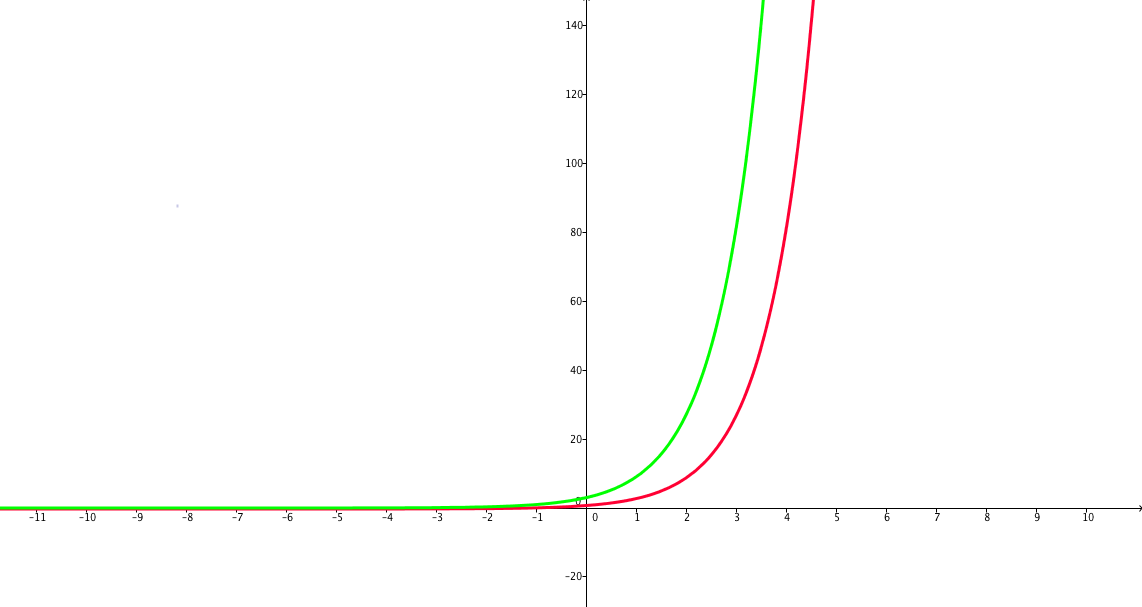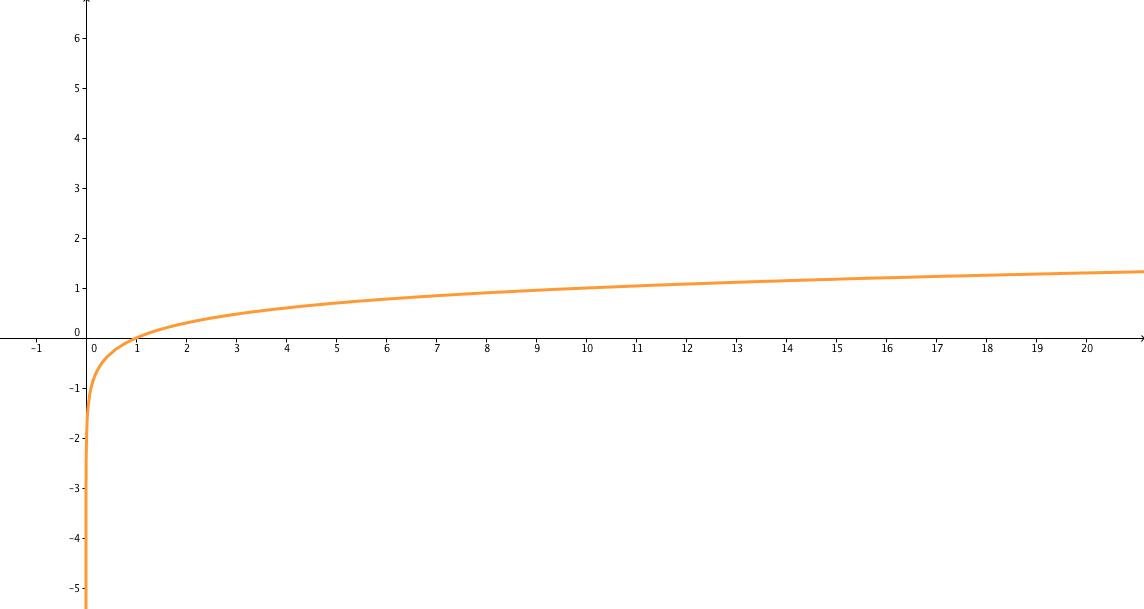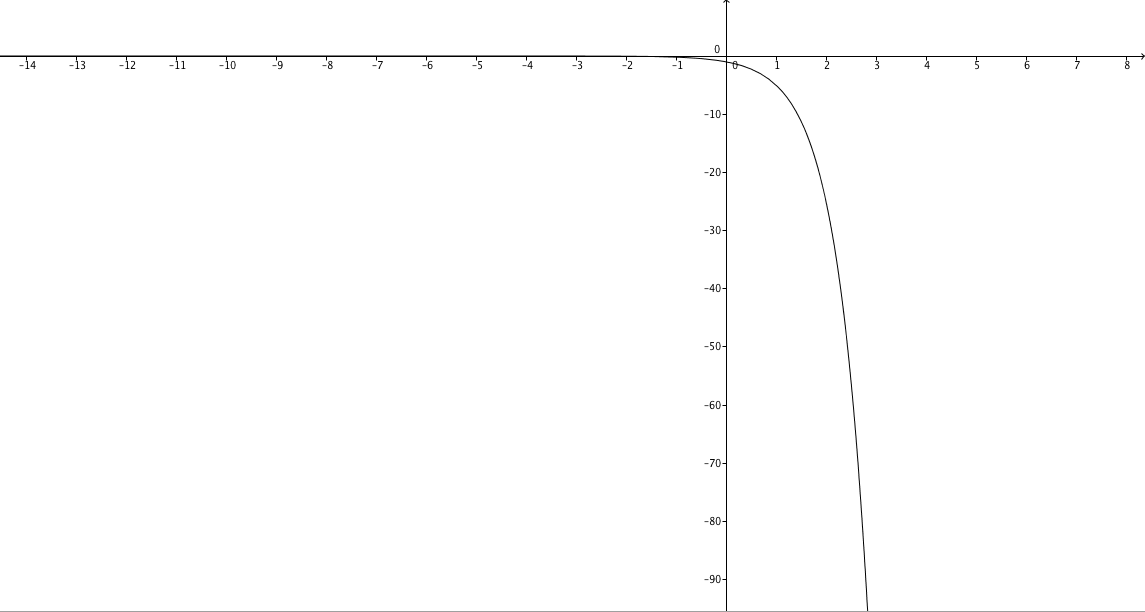Monotonically Increasing and Decreasing Functions: an Algebraic Approach

Article objectives

• The objective of this article is to introduce monotonically increasing and decreasing functions and their properties, specifically pertaining to exponential and logarithmic functions.
• Introduction

The groups of monotonically increasing and monotonically decreasing functions have some special properties. A monotonically increasing function is one that increases as $$x$$ does for all real $$x$$. A monotonically decreasing function, on the other hand, is one that decreases as $$x$$ increases for all real $$x$$. In particular, these concepts are helpful when studying exponential and logarithmic functions.

Monotonically Increasing Functions

The graphs of exponential and logarithmic functions will be crucial here. From them we can see a general rule:

If $$a > 1$$, then both of these functions are monotonically increasing:

$$f(x) = a^x$$ $$g(x) = \log_{a}(x)$$

By looking at a sufficient number of graphs, we can understand this. Over an interval on which a function is monotonically increasing (or decreasing), an output for the function will not occur more than once.

Example 1: Consider these two graphs. The red one is $$f(x) = 3^x$$ while the green one is $$g(x) = 3^{x + 1}$$:Notice that as $$x$$ is increasing, $$f(x)$$ is increasing. Now notice this algebraic manipulation:

$$g(x) = 3^{x + 1} = 3 \cdot 3^x = 3f(x)$$

Therefore, $$g(x)$$ is also monotonically increasing. You can multiply $$f(x)$$ by any positive constant, and the values of the new function will continue to grow at a faster or slower rate as $$x$$ increases.

Now we consider a logarithmic function.

Example 2: We will use an example involving the common logarithm. Let $$f(x) = \log_{10}(x)$$. A graph is shown below:The graph is curved upward where the function is defined (positive real numbers), in a way that it is always increasing. Any logarithm with a positive base will have a similar pattern.

Example 3: Explain why $$x = 0$$ is the only solution to $$3^x = 1$$.

Solution: Over the real numbers, the function $$f(x)$$ is monotonically increasing, so there can only be one solution, because no value of $$y$$ is achieved on the graph twice.

Monotonically Decreasing Functions

Monotonically decreasing functions are basically the opposite of monotonically increasing functions. As a result:

If $$f(x)$$ is a monotonically increasing function over some interval, then $$-f(x)$$ is a monotonically decreasing function over that same interval, and vice-versa.

Here is an example of a monotonically decreasing function.

Example 4: Consider the graph of $$f(x) = -5^x$$, shown below:The function $$5^x$$ is monotonically increasing, so $$f(x) = -5^x$$ must be monotonically decreasing, because wherever $$5^x$$ is increasing (everywhere), $$f(x)$$ is decreasing.

When a Function is not Monotonically Increasing or Decreasing

There are some functions that are not monotonically increasing nor monotonically decreasing. There are an infinite number of these functions, and they belong to many different groups.

Main Group 1: Constant Functions

These are straight lines, so they are not decreasing or decreasing.

Main Group 2: Absolute Value Functions

Functions surrounded by an absolute value sign are always nonnegative, but then all non-constant functions of this type will have a minimum. Therefore the function will alternate between increasing and decreasing as $$x$$ increases.

Main Group 3: Trigonometric Functions

Consider basic trigonometric functions such as $$\sin(x)$$, which move up and down, and thus do not exclusively increase or decrease.

Main Group 4: Functions with Discontinuities

A function cannot increase or decrease over any type of discontinuity, especially when the discontinuity is caused by an undefined value (i.e. $$x = 0$$ in $$f(x) = \frac{1}{x}$$).

Monotonically increasing and decreasing graphs can be identified by graphs, but this is not a very rigorous method. Still, it is good for students who do not have any calculus background. There are methods containing much more detail and rigor that involve calculus, related to the rate of change of the function as $$x$$ changes.